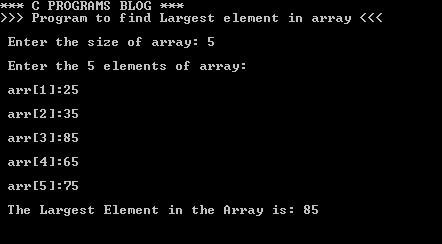# C PROGRAM TO FIND LARGEST / BIGGEST NUMBER IN ARRAY

### C PROGRAM TO FIND LARGEST / BIGGEST NUMBER IN ARRAY

```#include<stdio.h>
#define MAX 25 //maximum size of array is 25

main()
{
int i,n,LargeNo,arr[MAX];

printf("*** C PROGRAMS BLOG ***");
printf("\n>>> Program to find Largest element in array <<<\n");
printf("\n Enter the size of array: ");
scanf("%d",&n); //taking size of array as input from user
printf("\n Enter the %d elements of array: \n",n);

//taking input all the elements of array using for loop
for(i=0;i<n;i++)
{
printf("n arr[%d]:",i+1);
scanf("%d",&arr[i]);
}
LargeNo = arr;
for(i=0;i<n;i++)
{
if(arr[i]>LargeNo)
{
LargeNo = arr[i];
}
}
//printing the largest element in array
printf("n The Largest Element in the Array is: %d ",LargeNo);
getch();
}```

Explanation :

The above program is to find the largest element in the given array of elements. Here we are using declaring the maximum size of array as 25 using #define directive .

Sample Output :